# How is M2 (M squared) calculated in Physical Optics Propagation (POPD)?

• 0 replies
• 539 views

Userlevel 5+2
• Zemax Staff
• 108 replies

Many users might have questions how exactly M2 is calculated and let’s discover this in this post! We will first explain its equation and then show how you can reproduce it in OpticStudio.

The only way to get M2 is by using POPD operand in merit function. To calculated M2, the Data parameter must be either 25 (X direction) or 26 (Y direction). To use POPD operand, first define the settings on the POP analysis feature as desired, then press Save on the settings box.

More information about POPD can be found in Help file > The Optimize Tab (sequential ui mode) > Automatic Optimization Group > Merit Function Editor (automatic optimization group) > Optimization Operands by Category > Physical Optics Propagation (POP) Results

Now let’s start to see how it’s calculated. As can be found in Help file, we use the following equation to calculate: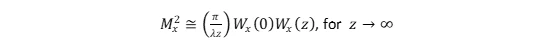where Wx(0) is the minimum beam size of the real beam and W(z) is the beam size at a large z position.

For the Wx(0), we use the beam width (POPD with Data = 23 or 24) at the position of the pilot beam's waist.

For the Wx(z) with z->inf, we use the beam width (POPD with Data = 23 or 24) at a large z. For example, we can use 1e5*Rayleigh Length for large z.

Let’s open the following example file to test.

{Zemax}\Samples\Sequential\Objectives\Double Gauss 28 degree field.zmx

Then we open POP analysis, click Reset and Save. Now any POPD you set in the merit function will use the settings you just saved.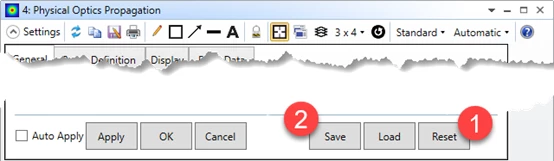As shown below, we can see the M2 is calculated as 3.992486 in both X and Y directions. It’s not surprised value at X and Y are same because this is an rotationally-symmetrical system.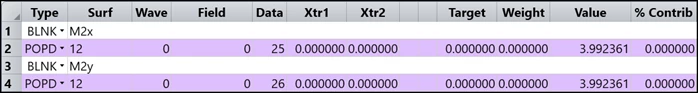Let’s check the pilot beam position and Rayleigh range using Data = 5 and 6.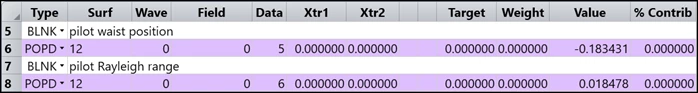Now add two surfaces in LDE to get position of pilot beam waist position and far field. The far field is calculated as 1e5 times of Rayleigh range. This can be any number as long as it’s large compared to Rayleigh range.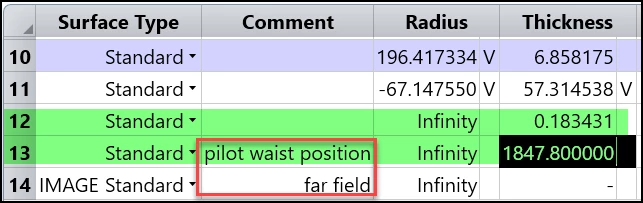The w(0) and w(z) is then calculated by using POPD with Data = 23 at surface 13 and surface 14.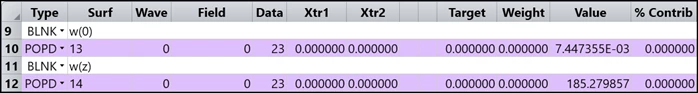Then we can calculate the M2 as shown below. M2 is at the final line. Note there is a little error if we compare line 28 below and line 2 above. This is because in POPD we use a more efficient way to calculate this. However, the concept is still same and we can see the difference very small between 3.992361 and 3.992554.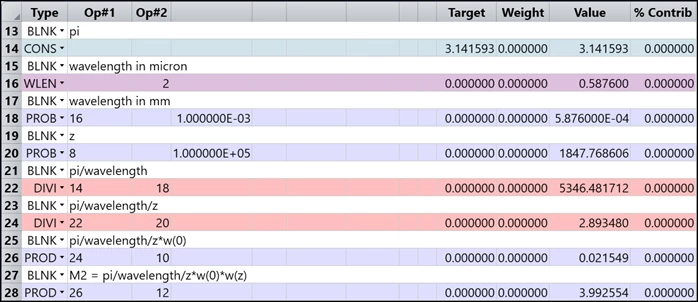The final file is attached!